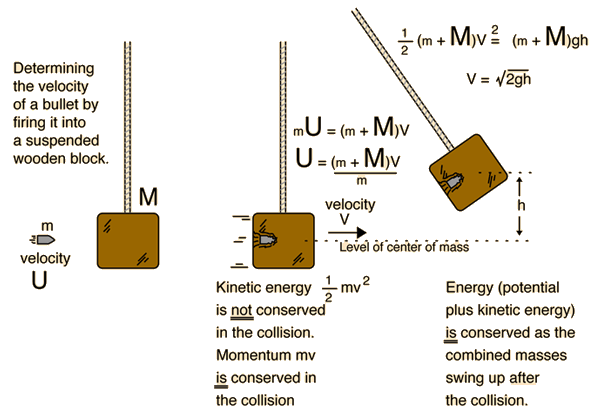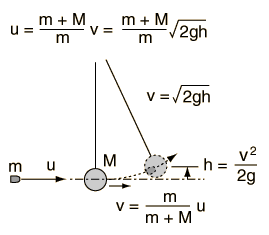# Ballistic Pendulum

The ballistic pendulum is a classic example of a dissipative collision in which conservation of momentum can be used for analysis, but conservation of energy during the collision cannot be invoked because the energy goes into inaccessible forms such as internal energy. After the collision, conservation of energy can be used in the swing of the combined masses upward, since the gravitational potential energy is conservative.Calculation
Index

Collision concepts

 HyperPhysics***** Mechanics R Nave
Go Back

# Ballistic PendulumIn the back courtyard of the munitions factory hung an old, scarred block of wood. As quality control for the cartridges coming off the assembly line, someone would regularly take a gun to the courtyard and fire a bullet into the block. Measuring the height of the swing revealed the speed of the bullet, but since the block was increasing in mass with the added bullets, the mass of the block had to be checked as well as the mass of the bullet being fired.

If a bullet of mass m = grams

and a muzzle velocity u = m/s = km/h = mi/h

is fired into a block of mass M = grams

then the velocity of the block and bullet after the impact would be

v = m/s = km/h = mi/h

and the combination of block and bullet would swing above its original height by an amount

h = m = cm = ft.

Comments on calculation: If a value for the velocity of the bullet, u, or either of the masses is entered, the velocity v after the collision and the height of swing is calculated. If either the velocity v after the collision or the height h is entered, then the other values will be calculated presuming the current values of the masses. A value of g = 9.8 m/s2 is assumed in the calculation.

 Further discussion
Index

Collision concepts

 HyperPhysics***** Mechanics R Nave
Go Back